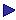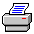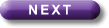Tutorial Contents Tutorial Eight: Relations- Reflexivity - Symmetry -Transitivity - Connectedness - Example of a tableau proof - Eqivalent Relations More Tutorials OneTwoThreeFourFiveSixConnectedness

Rxy is connected just if any two dots are connected by an arrow in at least one direction. That is:

Rxy is connected just if "x"y[¬x=y®[RxyÚRyx]]

or, equivalently,

Rxy is connected just if ¬\$x\$y[¬x=yÙ[¬RxyÙ¬Ryx]].

So,

Rxy is not connected just if \$x\$y[¬x=yÙ[¬RxyÙ¬Ryx]].

(For practice in seeing equivalences, note that ""x"y[¬x=y®[RxyÚRyx]]" is equivalent to "¬\$x¬¬\$y¬[¬x=y®[RxyÚRyx]]" (because ""xj" is equivalent to "¬\$j"), which is equivalent to "¬\$x\$y¬[¬x=y®[RxyÚRyx]]" (because "¬¬j" is equivalent to "j"), which is equivalent to "¬\$x\$y[¬x=yÙ¬[RxyÚRyx]]" (because "¬[j®y]" is equivalent to "[¬y]"), which is equivalent to "¬\$x\$y[¬x=yÙ[¬RxyÙ¬Ryx]]" (because "¬[jÚy]" is equivalent to "[¬¬y]").

Examples: "x>y" is connected in the domain of numbers; "¬x=y" is connected in every domain (because, of course, "x"y[¬x=y®[¬x=yÚ¬y=x]]); "¬x is older than y" is connected in every domain. On the other hand "x is older than y" is not connected in any domain which contains two things of the same age, or something that lacks an age.Print this page